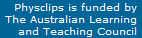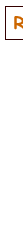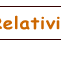# Friction, normal forces, contact forces: simple experiments

Friction and normal forces are components of contact forces, chosen for convenience in analysis. This is a supporting page to the multimedia tutorial on contact forces in Physclips. Its purpose is to allow the user to examine these experiments more closely.

### Coefficients of static and kinetic friction

This set of film clips shows a simple experiment designed to determine the coefficients of static and kinetic friction. The table top is believed to be vinyl. The lowest disc is mounted on a sample of artificial turf. The iron discs are masses originally used on a balance. Each has a mass of 1 kg. A spring balance is used to apply and to measure the horizontal force. In order to make short, simple film clips, the design does not include precise control of applied force. To determine limiting friction, the lateral force was gradually increased. The last frame before the load first moved is displayed as a still to estimate the applied horizontal force, which is assumed approximately equal to (minus one times) the limiting friction.
If the velocity is constant, then kinetic friction equals minus one times the applied horizontal force. Using the single step button and examining the video frames, we see that, after the first few frames of motion, the velocity is approximately constant. Again, a still is used to read the applied force.
 These experiments show that, for these two surfaces and over this range of forces, both kinetic friction Fk and limiting friction Fmax are approximately proportional to the weight and thus to the normal force. This allows us to define coefficients of kinetic and static friction for these surfaces, with approximate values μk = 0.43 and μs = 0.33. As is often the case for clean, dry surfaces, μk< μs. In other experiments shown briefly on the multimedia tutorial, we replaced the artificial turf with felt and then with nothing, giving us coefficients of μs = 0.38 for felt-vinyl and μs = 0.9 iron-vinyl in this geometry.

### Dependence on area of the limiting coefficient of static friction

The experiment shown at right is one that is easy to do in class. Increase the angle θ of an inclined plane slowly until an object begins to slide: we saw in Weight and Contact Forces that the sliding begins at a critical angle when tan θc  =  μs. It's harder to do, but the maximum angle at which the sliding object comes to rest has tan θ'c  =  μk.

The experiment illustrated uses a rubber (an eraser, for Americans) on an inclined plane, here a text book. By changing the orientation of the rubber, one can investigate the dependence of μs on the area of contact. It is important to clean the surfaces, to raise the inclined plane slowly and to do a number of repetitions, because the dependence is quite weak and may be masked by subtle variations in the surface. We did six repetitions and show here the smallest and largest angles for each geometry.

The simplicity of performing this experiment is an advantage, because this dependence of μs on the area is often hotly debated, especially by boys who have been persuaded by rubber companies to put fat wheels on cars. For these surfaces and geometries, μs is slightly smaller when the area is increased. The multimedia tutorial Weight and Contact Forces shows another variant where, at constant area, the load can be varied.

### Happy birthday, theory of relativity!

As of June 2005, relativity is 100 years old. Our contribution was Einstein Light: relativity in brief... or in detail. It explains the key ideas in a short multimedia presentation, which is supported by links to broader and deeper explanations.This work is licensed under a Creative Commons License.